##MSIPO技术圈 首页 IT技术 查看内容

# 算法学习 day23

2023-07-13

### 669. 修剪二叉搜索树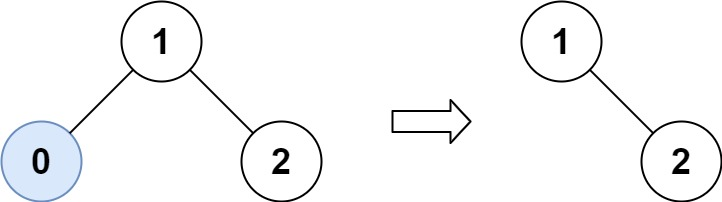``````输入：root = [1,0,2], low = 1, high = 2

``````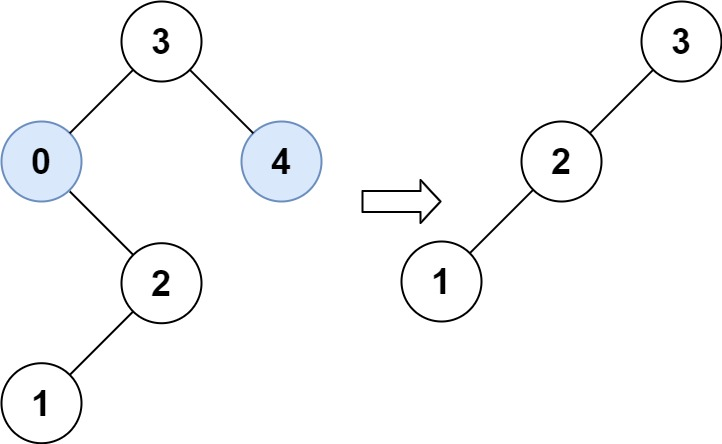``````输入：root = [3,0,4,null,2,null,null,1], low = 1, high = 3

``````

• 树中节点数在范围 `[1, 104]`
• `0 <= Node.val <= 104`
• 树中每个节点的值都是 唯一
• 题目数据保证输入是一棵有效的二叉搜索树
• `0 <= low <= high <= 104`

#### 递归解法

``````class Solution {
public TreeNode trimBST(TreeNode root, int low, int high) {
//终止条件
if(root == null) return root;
//单层循环逻辑
//当前值小于目标值，修剪左子树，返回右子树
if(root.val < low) return trimBST(root.right,low,high);
//反之
if(root.val > high) return trimBST(root.left,low,high);

//当前值符合范围，分别修建左右子树
root.left = trimBST(root.left,low,high);
root.right = trimBST(root.right,low,high);
return root;
}
}
``````

### 108.将有序数组转换为二叉搜索树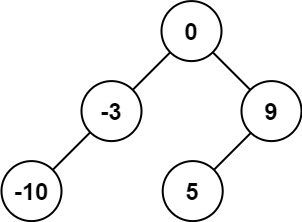``````输入：nums = [-10,-3,0,5,9]

``````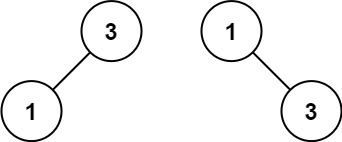``````输入：nums = [1,3]

``````

• `1 <= nums.length <= 104`
• `-104 <= nums[i] <= 104`
• `nums`严格递增 顺序排列

#### 递归

• 二叉搜索树按照中序（左根右）遍历，得到升序数组
• 通过中间件节点切分得到根节点即可
``````class Solution {
public TreeNode sortedArrayToBST(int[] nums) {
return sortedSubBST(nums,0,nums.length-1) ;
}
public TreeNode sortedSubBST(int[] nums,int start,int end) {
if(start>end) return null;
int mid = start + (end - start)/2;
TreeNode root = new TreeNode(nums[mid]);
root.left = sortedSubBST(nums,start,mid-1);
root.right = sortedSubBST(nums,mid+1,end);
return root;
}
}
``````

### 538.将二叉树搜索树转化为累加树

• 节点的左子树仅包含键 小于 节点键的节点。
• 节点的右子树仅包含键 大于 节点键的节点。
• 左右子树也必须是二叉搜索树。

**注意：**本题和 1038: https://leetcode-cn.com/problems/binary-search-tree-to-greater-sum-tree/ 相同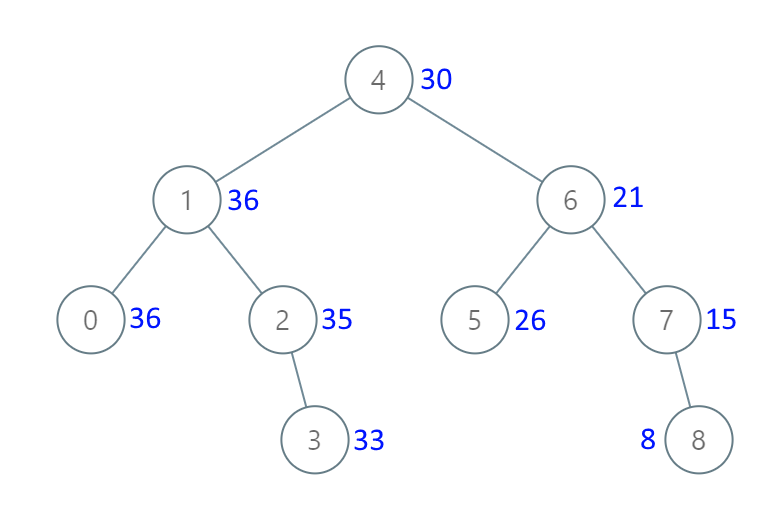``````输入：[4,1,6,0,2,5,7,null,null,null,3,null,null,null,8]

``````

``````输入：root = [0,null,1]

``````

``````输入：root = [1,0,2]

``````

``````输入：root = [3,2,4,1]

``````

• 树中的节点数介于 `0``104` 之间。
• 每个节点的值介于 `-104``104` 之间。
• 树中的所有值 互不相同
• 给定的树为二叉搜索树。

#### 递归

• 题意中的累加和理解为从大到小累加得出
• 中序遍历的结果为从小到大，即升序，要想得到降序的数组，使用和中序相反的顺序遍历（右根左）
• 此时根据递归三部曲
• 参数和返回值为根节点
• 终止条件，节点为null
• 单层递归逻辑
• 先遍历右子树，累加和->给右子节点赋值
• 计算节点和
• 最后遍历左子树
``````class Solution {
int sum = 0 ;
public TreeNode convertBST(TreeNode root) {
if(root == null)return null;

convertBST(root.right);
sum += root.val;
root.val = sum;
convertBST(root.left);

return root;
}
}
``````